# Sudoku using POCS and Sparsity¶

Important: Please read the installation page for details about how to install the toolboxes. $\newcommand{\dotp}{\langle #1, #2 \rangle}$ $\newcommand{\enscond}{\lbrace #1, #2 \rbrace}$ $\newcommand{\pd}{ \frac{ \partial #1}{\partial #2} }$ $\newcommand{\umin}{\underset{#1}{\min}\;}$ $\newcommand{\umax}{\underset{#1}{\max}\;}$ $\newcommand{\umin}{\underset{#1}{\min}\;}$ $\newcommand{\uargmin}{\underset{#1}{argmin}\;}$ $\newcommand{\norm}{\|#1\|}$ $\newcommand{\abs}{\left|#1\right|}$ $\newcommand{\choice}{ \left\{ \begin{array}{l} #1 \end{array} \right. }$ $\newcommand{\pa}{\left(#1\right)}$ $\newcommand{\diag}{{diag}\left( #1 \right)}$ $\newcommand{\qandq}{\quad\text{and}\quad}$ $\newcommand{\qwhereq}{\quad\text{where}\quad}$ $\newcommand{\qifq}{ \quad \text{if} \quad }$ $\newcommand{\qarrq}{ \quad \Longrightarrow \quad }$ $\newcommand{\ZZ}{\mathbb{Z}}$ $\newcommand{\CC}{\mathbb{C}}$ $\newcommand{\RR}{\mathbb{R}}$ $\newcommand{\EE}{\mathbb{E}}$ $\newcommand{\Zz}{\mathcal{Z}}$ $\newcommand{\Ww}{\mathcal{W}}$ $\newcommand{\Vv}{\mathcal{V}}$ $\newcommand{\Nn}{\mathcal{N}}$ $\newcommand{\NN}{\mathcal{N}}$ $\newcommand{\Hh}{\mathcal{H}}$ $\newcommand{\Bb}{\mathcal{B}}$ $\newcommand{\Ee}{\mathcal{E}}$ $\newcommand{\Cc}{\mathcal{C}}$ $\newcommand{\Gg}{\mathcal{G}}$ $\newcommand{\Ss}{\mathcal{S}}$ $\newcommand{\Pp}{\mathcal{P}}$ $\newcommand{\Ff}{\mathcal{F}}$ $\newcommand{\Xx}{\mathcal{X}}$ $\newcommand{\Mm}{\mathcal{M}}$ $\newcommand{\Ii}{\mathcal{I}}$ $\newcommand{\Dd}{\mathcal{D}}$ $\newcommand{\Ll}{\mathcal{L}}$ $\newcommand{\Tt}{\mathcal{T}}$ $\newcommand{\si}{\sigma}$ $\newcommand{\al}{\alpha}$ $\newcommand{\la}{\lambda}$ $\newcommand{\ga}{\gamma}$ $\newcommand{\Ga}{\Gamma}$ $\newcommand{\La}{\Lambda}$ $\newcommand{\si}{\sigma}$ $\newcommand{\Si}{\Sigma}$ $\newcommand{\be}{\beta}$ $\newcommand{\de}{\delta}$ $\newcommand{\De}{\Delta}$ $\newcommand{\phi}{\varphi}$ $\newcommand{\th}{\theta}$ $\newcommand{\om}{\omega}$ $\newcommand{\Om}{\Omega}$

This numerical tour explores the use of numerical schemes to solve the Sudoku game.

This tour was written by <http://lcav.epfl.ch/~lu/ Yue M. Lu> and <http://www.ceremade.dauphine.fr/~peyre/ Gabriel Peyr >.

The idea of encoding the Sudoku rule using a higer dimensional lifting, linear constraints and binary constraint is explained in:

Andrew C. Bartlett, Amy N. Langville, An Integer Programming Model for the Sudoku Problem, The Journal of Online Mathematics and Its Applications, Volume 8. May 2008.

The idea of removing the binary constraint and using sparsity constraint is exposed in:

P. Babu, K. Pelckmans, P. Stoica, and J. Li, Linear Systems, Sparse Solutions, and Sudoku, IEEE Signal Processing Letters, vol. 17, no. 1, pp. 40-42, 2010.

This tour elaborarates on these two ideas. In particular it explains why $L^1$ minimization is equivalent to a POCS (projection on convex sets) method to find a feasible point inside a convex polytope.

In :
addpath('toolbox_signal')


## Game Encoding and Decoding¶

The basic idea is to use a higher dimensional space of size |(n,n,n)| to represent a Sudoku matrix of size |(n,n)|. In this space, the arrays are constrained to have binary entries.

Size of the Sudoku. This number must be a square.

In :
n = 9;


Create a random integer matrix with entries in 1...9.

In :
x = floor(rand(n)*n)+1;


Comparison matrix used for encoding to binary format.

In :
U = repmat( reshape(1:n, [1 1 n]), n,n );


Encoding in binary format.

In :
encode = @(x)double( repmat(x, [1 1 n])==U );
X = encode(x);


The resulting matrix has binary entries and has size |(n,n,n)|. One has |x(i,j)=k| if |X(i,j,k)=1| and |X(i,j,l)=0| for |l~=k|.

Decoding from binary format. One use a |min| to be able to recover even if the matrix |X| is not binary (because of computation errors).

In :
[tmp,x1] = min( abs(X-1), [], 3 );


Show that decoding is ok.

In :
disp(['Should be 0: ' num2str(norm(x-x1,'fro')) '.']);

Should be 0: 0.


## Encoding Constraints¶

For |X| to be a valid encoded matrix, it should be binary and satifies that each |X(i,j,:)| contains only a single |1|, which can be represented using a linear contraint |Aenc*X(:)=1|.

Now we construct one encoding constraint.

In :
i = 4; j = 4;
Z = zeros(n,n,n);
Z(i,j,:) = 1;


Add this constraint to the encoding constraint matrix.

In :
Aenc = [];
Aenc(end+1,:) = Z(:)';


Show that constraint is satisfied.

In :
disp(['Should be 1: ' num2str(Aenc*X(:)) '.']);

Should be 1: 1.


Exercise 1

Build the encoding matrix |Aenc|. Display it.

In :
exo1()In :
%% Insert your code here.


Show that constraint |Aenc*X(:)=1| is satisfied.

In :
disp(['Should be 0: ' num2str(norm(Aenc*X(:)-1)) '.']);

Should be 0: 0.


## Sudoku Rules Constraints¶

In a Sudoku valid matrix |x|, each column, row and sub-square of |x| should contains all the values in 0...n. This can be encoded on the high dimensional |X| using linear constraints |ArowX=1|, |AcolX=1| and |Ablock*X=1|.

A valid Sudoku matrix.

In :
x = [8 1 9 6 7 4 3 2 5;
5 6 3 2 8 1 9 4 7;
7 4 2 5 9 3 6 8 1;
6 3 8 9 4 5 1 7 2;
9 7 1 3 2 8 4 5 6;
2 5 4 1 6 7 8 9 3;
1 8 5 7 3 9 2 6 4;
3 9 6 4 5 2 7 1 8;
4 2 7 8 1 6 5 3 9]

x =

8     1     9     6     7     4     3     2     5
5     6     3     2     8     1     9     4     7
7     4     2     5     9     3     6     8     1
6     3     8     9     4     5     1     7     2
9     7     1     3     2     8     4     5     6
2     5     4     1     6     7     8     9     3
1     8     5     7     3     9     2     6     4
3     9     6     4     5     2     7     1     8
4     2     7     8     1     6     5     3     9



Encode it in binary format.

In :
X = encode(x);


Select the index of the entries of a row.

In :
i=3; k=5;
Z = zeros(n,n,n);
Z(i,:,k) = 1;


Fill the first entries of the row matrix.

In :
Arow = [];
Arow(end+1,:) = Z(:)';


Show that constraint is satisfied.

In :
disp(['Should be 1: ' num2str(Arow*X(:)) '.']);

Should be 1: 1.


Exercise 2

Build the full row matrix |Arow|. Display it.

In :
exo2()In :
%% Insert your code here.


Show that constraint |Arow*X(:)=1| is satisfied.

In :
disp(['Should be 0: ' num2str(norm(Arow*X(:)-1)) '.']);

Should be 0: 0.


Exercise 3

Build the full column matrix |Acol|. Display it.

In :
exo3()In :
%% Insert your code here.


Show that constraint |Acol*X(:)=1| is satisfied.

In :
disp(['Should be 0: ' num2str(norm(Acol*X(:)-1)) '.']);

Should be 0: 0.


Now we proceed to block constraints.

Size of a block.

In :
p = sqrt(n);


The upper left square should contain all numbers in |{1,...,n}|.

In :
k = 1;
Z = zeros(n,n,n);
Z(1:p,1:p,k) = 1;


Add it as the first row of the block constraint matrix.

In :
Ablock = [];
Ablock(end+1,:) = Z(:)';


Show that constraint is satisfied.

In :
disp(['Should be 1: ' num2str(Ablock*X(:)) '.']);

Should be 1: 1.


Exercise 4

Create the full block matrix. Display it.

In :
exo4()In :
%% Insert your code here.


Show that constraint |Ablock*X(:)=1| is satisfied.

In :
disp(['Should be 0: ' num2str(norm(Ablock*X(:)-1)) '.']);

Should be 0: 0.


## Inpainting Constraint¶

A Sudoku game asks to fill the missing entries of a partial Sudoku matrix |x1| to obtain a full Sudoku matrix |x|.

The fact that for each available entry |(i,j)| on must have |x(i,j)=x1(i,j)| can be encoded using a linear constraint.

Load a Sudoku with missing entries, that are represented as 0. This is an easy grid.

In :
x1 = [0 1 0 0 0 0 3 0 0;
0 0 3 0 8 0 0 4 0;
7 0 2 0 0 3 0 0 1;
0 3 0 9 4 0 1 0 0;
9 0 0 0 0 0 0 0 6;
0 0 4 0 6 7 0 9 0;
1 0 0 7 0 0 2 0 4;
0 9 0 0 5 0 7 0 0;
0 0 7 0 0 0 0 3 0];


Retrieve the indexes of the available entries.

In :
[I,J] = ind2sub( [n n], find(x1(:)~=0) );
v = x1(x1(:)~=0);


Create a vector corresponding to the constraint that |x1(I(i),J(i))==v(i)|.

In :
i = 1;
Z = zeros(n,n,n);
Z(I(i), J(i), v(i)) = 1;


Fill the first entries of the row matrix.

In :
Ainp = [];
Ainp(end+1,:) = Z(:)';


Exercise 5

Build the full inpainting matrix |Ainp|. Display it.

In :
exo5()In :
%% Insert your code here.


Show that constraint |Ainp*X1(:)=1| is satisfied.

In :
X1 = encode(x1);
disp(['Should be 0: ' num2str(norm(Ainp*X1(:)-1)) '.']);

Should be 0: 0.


## Solving the Sudoku by Binary Integer Programming¶

The whole set of constraints can be written |A*X(:)=1|, where the matrix |A| is defined as a concatenation of all the constraint matrices.

In :
A = [Aenc; Arow; Acol; Ablock; Ainp];


Pre-compute the pseudo-inverse of A.

In :
pA = pinv(A);


If the Sudoku game has an unique solution |x|, then the corresponding lifted vector |X| is the only solution to |A*X(:)=1| under the constraint that |X| is binary.

This is the idea proposed in:

Andrew C. Bartlett, Amy N. Langville, An Integer Programming Model for the Sudoku Problem, The Journal of Online Mathematics and Its Applications, Volume 8. May 2008.

Unfortunately, solving a linear system under binary constraints is difficult, in fact solving a general integer program is known to be NP-hard. It means that such a method is very slow to solve Sudoku for large |n|.

One can use branch-and-bounbs methods to solve the binary integer program, but this might be slow. One can use for instance the command |bintprog| of Matlab (optimization toolbox), with an arbitrary objective function (since one wants to solve a feasability problem, no objective is needed).

Exercise 6

Implement the Soduku solver using an interger linear programming algorithm.

In :
exo6()

In :
%% Insert your code here.


## Removing the Binary Constraint¶

If one removes the binary constraint, one simply wants to compute a solution to the linear system |A*X(:)=1|. But unfortunately it has an infinite number of solutions (and the set of solutions is not bounded).

It is thus unlikely that chosing a solution at random will work, but let's try it by projecting any vector on the constraint |A*X(:)=1|.

First define the orthogonal projector on the constraint |{X \ A*X(:)=1}|.

In :
projector = @(u)reshape( u(:) - pA*(A*u(:)-1), [n n n]);


We project an arbitrary vector (that does not satisfy the constraint) onto the constraint |A*X(:)=1|.

In :
Xproj = projector( zeros(n,n,n) );


Check that |Xproj| projects onto itself because it satisfies the constraints.

In :
d = projector(Xproj)-Xproj;
disp(['Should be 0: ' num2str(norm(d(:), 'fro')) '.']);

Should be 0: 4.1665e-14.


Plot the histogrtam of the entries of |Xproj|. As you can see, they are not binary, meaning that the binary constraint is violated.

In :
clf;
hist(Xproj(:), 30);
axis('tight');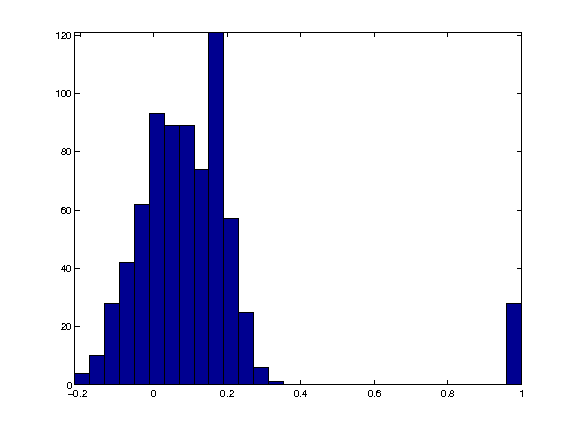It is thus not a solution to the Sudoku problem. We emphasize this by counting the number of violated constraints after decoding / re-encoding.

In :
[tmp,xproj] = min( abs(Xproj-1), [], 3 );
Xproj1 = encode(xproj);
disp(['Number of violated constraints: ' num2str(sum(A*Xproj1(:)~=1)) '.']);

Number of violated constraints: 119.


## Solving the Sudoku by Projection on Convex Sets¶

A way to improve the quality of the result is to find a vector that satisfies both |AX(:)=1| and |0<=X<=1|. Note that this last constraint can be modified to |X>=0| because of the fact that the entries of |X(i,j,:)| must sum to 1 because of |AX(:)|.

A way to find a point inside this polytope |P = {X \ A*X(:)=1 and X>=0}| is to start from a random initial guess.

In :
Xproj = zeros(n,n,n);


And iteratively project on each constraint. This corresponds to the POCS algorithm to find a feasible point into the (non empty) intersection of convex sets.

In :
Xproj = max( projector(Xproj),0 );


Exercise 7

Perform iterative projections (POCS) on the two constraints |AXproj(:)=1| and |Xproj>=0|. Display the decay of the error |norm(AXproj(:)-1)| in logarithmic scale.

In :
exo7()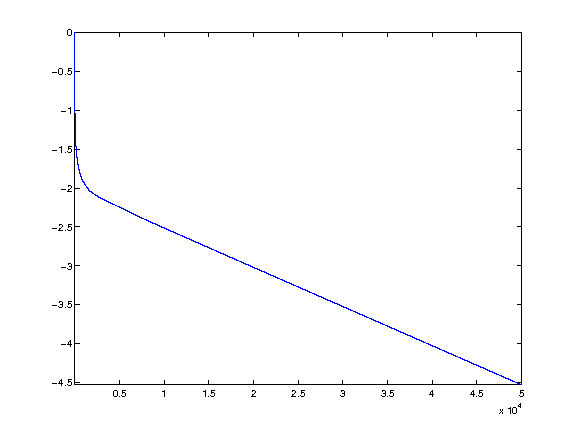In :
%% Insert your code here.


Display the histogram of the recovered values.

In :
clf;
hist(Xproj(:), 30);
axis('tight');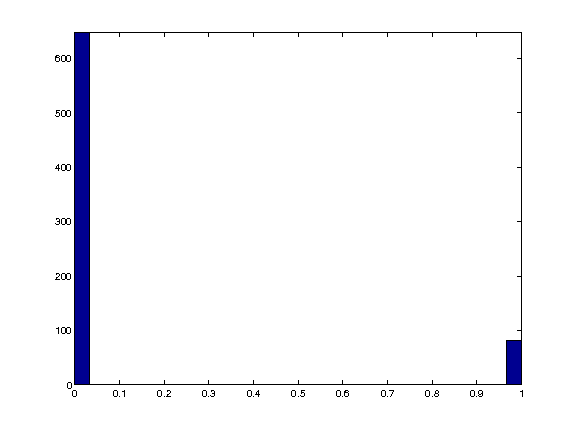As you can see, the resulting vector is (nearly, up to convergence errors of the POCS) a binary one, meaning that it is actually the (unique) solution to the Sudoku problem.

We check this by counting the number of violated constraints after decoding and re-encoding.

In :
[tmp,xproj] = min( abs(Xproj-1), [], 3 );
Xproj1 = encode(xproj);
disp(['Number of violated constraints: ' num2str(sum(A*Xproj1(:)~=1)) '.']);

Number of violated constraints: 0.


Exercise 8

Prove (numerically) that for this grid, the polytope of constraints |P={X \ A*X(:)=1 and X>=0}| is actually reduced to a singleton, which is the solution of the Sudoku problem.

In :
exo8()

In :
%% Insert your code here.


Unfortunately, this is not always the case. For more difficult grids, |P| might not be reduced to a singleton.

This is a difficult grid.

In :
x1 = [0 0 3 0 0 9 0 8 1;
0 0 0 2 0 0 0 6 0;
5 0 0 0 1 0 7 0 0;
8 9 0 0 0 0 0 0 0;
0 0 5 6 0 1 2 0 0;
0 0 0 0 0 0 0 3 7;
0 0 9 0 2 0 0 0 8;
0 7 0 0 0 4 0 0 0;
2 5 0 8 0 0 6 0 0];


Exercise 9

Try the iterative projection on convexs set (POCS) method on this grid (remember that you need to re-define |A| and |pA|). What is your conclusion ? ill the constraint matrix OCS heck wether this is a valid solution.

In :
exo9()

Number of violated constraints: 20.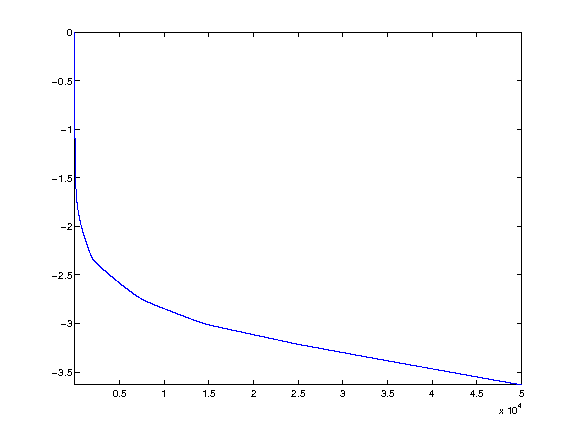In :
%% Insert your code here.


## Decoding Using L1 Sparsity¶

The true solution has exactly |n^2| non zero entries, while a feasible point within the convex polytope |P| is usually not as sparse.

Compute the sparsity of a projected vector.

In :
Xproj = projector( zeros(n,n,n) );
disp(['Sparsity: ' num2str(sum(Xproj(:)~=0)) ' (optimal: ' num2str(n*n) ').']);

Sparsity: 729 (optimal: 81).


One can prove that any solution to |AX(:)=1| has more than |n^2| non zeros, and that the true Sudoku solution is the unique solution to |AX(:)=1| with |n^2| entries.

One can thus (in principle) solve the Sudoku by finding the solution to |A*X(:)=1| with minimal L0 norm.

Unfortunately, solving this problem is known to be in some sense NP-hard.

A classical method to approximate the solution to the minimum L0 norm problem it to replace it by a minimum L1 norm solution, which can be computed with polynomial time algorithms.

This idea is put forward in:

P. Babu, K. Pelckmans, P. Stoica, and J. Li, Linear Systems, Sparse Solutions, and Sudoku, IEEE Signal Processing Letters, vol. 17, no. 1, pp. 40-42, 2010.

The L1 norm of the Sudoku solution is |n^2|. The L1 norm of a projected vector is usually larger.

In :
disp(['L1 norm: ' num2str(norm(Xproj(:),1)) ' (optimal: ' num2str(n*n) ').']);

L1 norm: 102.7585 (optimal: 81).


Unfortunately, all the vectors in the (bouned) polytope |A*X(:)=1| and |X>=0| has the same L1 norm, equal to |81|.

This shows that the L1 minimization has the same properties as the POCS algorithm. They work if and only if the polytope is reduced to a single point.

Nevertheless, one can compute the solution with minimum L1 norm which corresponds to the Basis pursuit problem. This problem is equivalent to a linear program, and can be solved using standard interior points method (other algorithms, such as Douglas-Rachford could be used as well).

Define a shortcut for the resolution of basis pursuit.

In :
solvel1 = @(A)reshape(perform_solve_bp(A, ones(size(A,1),1), n^3, 30, 0, 1e-10), [n n n]);


Solve the L1 minimization.

In :
Xbp = solvel1(A);


Compute the L1 norm of the solution, to check that it is indeed equal to the minimal possible L1 norm |n^2|.

In :
disp(['L1 norm: ' num2str(norm(Xbp(:),1)) ' (optimal: ' num2str(n*n) ').']);

L1 norm: 81 (optimal: 81).


Unfortunately, on this difficult problem, similarely to POCS, the L1 method does not works.

In :
[tmp,xbp] = min( abs(Xbp-1), [], 3 );
Xbp1 = encode(xbp);
disp(['Number of violated constraints: ' num2str(sum(A*Xbp1(:)~=1)) '.']);

Number of violated constraints: 16.


## Decoding Using more Aggressive Sparsity¶

Since the L1 norm does not perform better than POCS, it is tempting to use a more agressive sparsity measure, like |L^alpha| norm for |alpha<1|.

This leads to non-convex problems, and one can compute a (not necessarily globally optimal) local minimum.

An algorithm to find a local minimum of the energy is the reweighted L1 minimization, described in:

E. J. Cand s, M. Wakin and S. Boyd, Enhancing sparsity by reweighted l1 minimization, J. Fourier Anal. Appl., 14 877-905.

This idea is introduced in the paper:

P. Babu, K. Pelckmans, P. Stoica, and J. Li, Linear Systems, Sparse Solutions, and Sudoku, IEEE Signal Processing Letters, vol. 17, no. 1, pp. 40-42, 2010.

At each iteration of the algorithm, one minimizes

$$min \sum 1/u_k |x_k|$$

subject to $$A x=1$$

The weights are then updated as

$$u_k=|x_k|^{1-\alpha} + \epsilon$$

The weighted L1 minimization can be recasted as a traditional L1 minimization using a change of variables.

$$min |y|_1$$

subject to $$A diag(u) y=1$$ and $$x_k = y_k u_k$$

Set the target |alpha|, that should be in |0<=alpha<=1|.

In :
alpha = 0;


Set the regularisation parameter |epsilon|, that avoids a division by zero.

In :
epsilon = 0.1;


Initial set of weights.

In :
u = ones(n,n,n);


Solve the weighted L1 minimization.

In :
Xrw = solvel1( A*diag(u(:)) ) .* u;


Update the weights.

In :
u = (abs(Xrw).^(1-alpha)+epsilon);


Exercise 10

Compute the solution using the reweighted L1 minimization. Track the evolution of the number of invalidated constraints as the algorithm iterates.

In :
exo10()

Number of violated constraints: 0.

In :
%% Insert your code here.


Display the histogram.

In :
hist(Xrw(:), 30);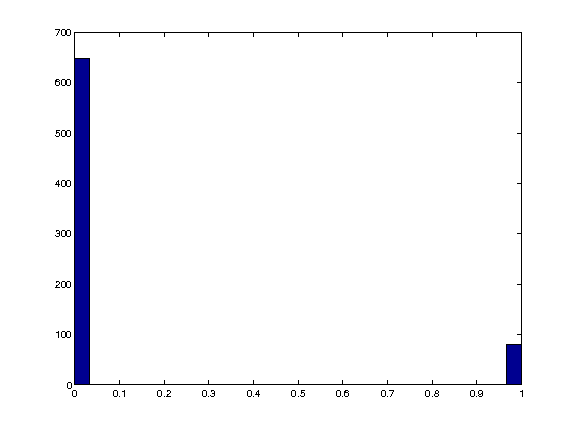While reweighting L1 works for reasonnably complicated Sudoku, it might fail on very difficult one.

This is the Al Escargot puzzle, believed to be the hardest Sudoku available.

In :
x1 = [1 0 0 0 0 7 0 9 0;
0 3 0 0 2 0 0 0 8;
0 0 9 6 0 0 5 0 0;
0 0 5 3 0 0 9 0 0;
0 1 0 0 8 0 0 0 2;
6 0 0 0 0 4 0 0 0;
3 0 0 0 0 0 0 1 0;
0 4 0 0 0 0 0 0 7;
0 0 7 0 0 0 3 0 0];


Exercise 11

Try reweighted L1 on this puzzle. ill matrix. olve

In :
exo11()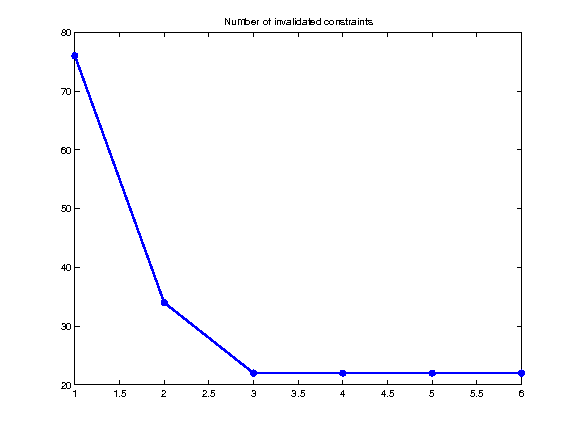In :
%% Insert your code here.


Exercise 12

Try other sparsity-enforcing minimization methods, such as Orthogonal Matching Pursuit (OMP), or iterative hard thresholding.

In :
exo12()

In :
%% Insert your code here.


Exercise 13

Try the different methods of this tour on a large number of Sudokus.

In :
exo13()

In :
%% Insert your code here.


Exercise 14

Try the different methods of this tour on larger Sudokus, for |n=4,5,6|.

In :
exo14()

In :
%% Insert your code here.